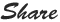# PHP Float Data type : How to write float in PHP?

PHP Float Data Type :

Float is number with decimals, any number with decimal point can be considered as float datatype.

Below example have \$f as float. and the function PHP var_dump() returns the value value of float.

Syntax:

<!DOCTYPE html>
<html>
<body>

<?php
\$f = 85.969;
var_dump(\$f);
?>

</body>
</html>

Output :

float(85.969)

--------------------